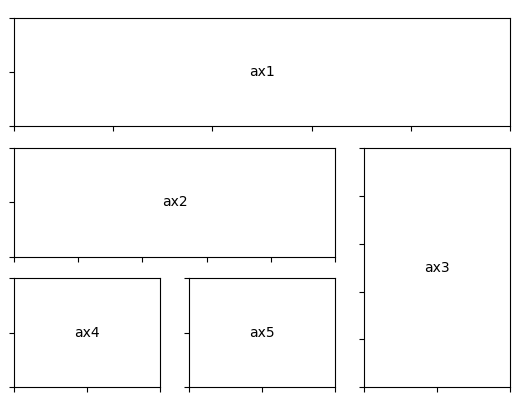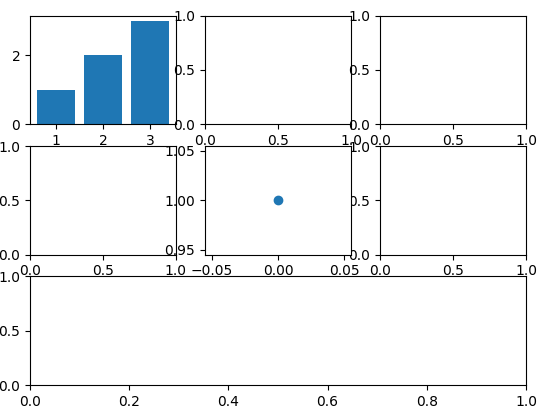## 函数概述

`subplot2grid`函数的功能是在特定网格位置中添加一个子图。

`ax = subplot2grid((nrows, ncols), (row, col), rowspan, colspan)`

• `shape`：定义子图网格的行数和列数。整数二元组，`(nrows, ncols)`
• `loc`：定义新增子图在子图网格中的位置（行、列索引）。整数二元组，`(nrow, ncol)`
• `rowspan`：定义新增子图向下跨越的行数。可选参数。整数，默认值为`1`
• `colspan`：定义新增子图向右跨越的列数。可选参数。整数，默认值为`1`
• `fig`：用于设置添加子图的`Figure`对象。可选参数。`Figure`对象。默认值为当前`Figure`对象。
• `subplot_kw` ：用于向创建子图时的底层函数 `~matplotlib.figure.Figure.add_subplot`传递关键字参数。可选参数。字典。
• `**kwargs`：用于向`~.Figure.add_subplot`传递的关键字参数。可选参数。

`.axes.SubplotBase`实例，或其他`~.axes.Axes`的子类实例。

## 函数原理

`subplot2grid`函数其实是`fig.add_subplot`方法的封装。源码为：

``````def subplot2grid(shape, loc, rowspan=1, colspan=1, fig=None, **kwargs):

if fig is None:
fig = gcf()

rows, cols = shape
gs = GridSpec._check_gridspec_exists(fig, rows, cols)

subplotspec = gs.new_subplotspec(loc, rowspan=rowspan, colspan=colspan)
bbox = ax.bbox
axes_to_delete = []
for other_ax in fig.axes:
if other_ax == ax:
continue
if bbox.fully_overlaps(other_ax.bbox):
axes_to_delete.append(other_ax)
for ax_to_del in axes_to_delete:
delaxes(ax_to_del)

return ax
``````

``````fig = gcf()
``````

## 案例：简易应用

``````import matplotlib.pyplot as plt

def annotate_axes(fig):
for i, ax in enumerate(fig.axes):
ax.text(0.5, 0.5, "ax%d" % (i+1), va="center", ha="center")
ax.tick_params(labelbottom=False, labelleft=False)

fig = plt.figure()
ax1 = plt.subplot2grid((3, 3), (0, 0), colspan=3)
ax2 = plt.subplot2grid((3, 3), (1, 0), colspan=2)
ax3 = plt.subplot2grid((3, 3), (1, 2), rowspan=2)
ax4 = plt.subplot2grid((3, 3), (2, 0))
ax5 = plt.subplot2grid((3, 3), (2, 1))

annotate_axes(fig)

plt.show()
``````## 案例：混合应用`subplot`、`subplots`、`subplot2grid`函数

``````import matplotlib.pyplot as plt

# 添加3行3列子图9个子图
fig, axes = plt.subplots(3, 3)
# 为第1个子图绘制图形
axes[0, 0].bar(range(1, 4), range(1, 4))
# 使用subplot函数为第5个子图绘制图形
plt.subplot(335)
plt.plot(1,'o')
# 使用subplot2grid函数将第三行子图合并为1个
plt.subplot2grid((3,3),(2,0),colspan=3)
# 返回值为Axes对象列表，元素个数为7
print(fig.axes)
plt.show()
``````## `subplot`、`subplot2grid`函数之间的比较

`subplot`函数和`subplot2grid`函数极其相似。

• 都可以向当前图像中添加一个子图。
• 返回值也都是一个`Axes`实例。
• 都可以指定子图在子图网格中的位置。

• `subplot`函数指定位置的方法相对比较隐晦，比如子图位置重叠时，隐含删除被覆盖子图。
• `subplot2grid`函数借助`gridspec`指定位置时相对清晰。

``````import matplotlib.pyplot as plt

# 绘制1行2列子图中的第1个子图
plt.subplot(121,facecolor='r')
# 绘制2行2列子图中的第2个子图
plt.subplot(222,facecolor='g')
# 绘制2行2列子图中的第4个子图
plt.subplot(224,facecolor='b')

plt.show()
``````

``````import matplotlib.pyplot as plt

# 绘制1行2列子图中的第1个子图
plt.subplot2grid((2,2),(0,0),rowspan=2,facecolor='r')
# 绘制2行2列子图中的第2个子图
plt.subplot(222,facecolor='g')
# 绘制2行2列子图中的第4个子图
plt.subplot(224,facecolor='b')

plt.show()
``````

### linux修改mysql配置文件_忘记MySQL密码了！？莫慌~-mysql教程-程序员宅基地

mysql教程栏目带大家解决忘记MySQL密码问题。在安装完 MySQL 或者是在使用 MySQL 时，最尴尬的就是忘记密码了，墨菲定律也告诉我们，如果一件事有可能出错，那么它一定会出错。那如果我们不小心忘记了 MySQL 的密码，该如何处理呢？别着急，本文教你一招搞定。1.修改 MySQL 配置文件首先我们需要打开 MySQL 的配置文件，如果是 Windows 系统的话，MySQL 的配置文件...

### validate无效，表单验证触发的修改_表单验证validate未触发-程序员宅基地

validate无效，鼠标失去焦点没有触发表单验证，表单验证触发的修改如果只是单纯的鼠标失去焦点没有触发validate表单验证，有可能是validate的默认验证触发方式的问题，默认触发方式为 onsumit 是提交时触发表单验证，只有在表单提交时触发表单验证。这种方式有时无返满足需求，可以开启其他的触发方式或关闭触发方式，方式如下：验证的触发方式有onsubmit，onfocusout，onkeyup，onclick，focusInvalid，用onfocusout为例：\$("表单的选择器"_表单验证validate未触发

_性质legesgue积分

### ubuntu 桌面版搭建qt环境的记录_ubuntu qt-程序员宅基地

linux下的qt搭建_ubuntu qt

### Qt中QTableWidget用法-程序员宅基地

Qt中QTableWidget用法

### JVM篇 -- JDK安装目录下的所有文件说明_jdk根目录全是文件-程序员宅基地

JDK安装目录下的文件说明1.bin该路径下存放了JDK的各种工具命令，常用的javac、java等命令就放在该路径下。基本工具这些工具是JDK的基础，用这些工具来编写应用程序。 工具名称 用途 javac.exe Java语言编译器 java.exe Java应用程..._jdk根目录全是文件

### PreparedStatement与SQL批处理-程序员宅基地

1、服务器如何执行SQL语句？　　当服务器接收到一条SQL语句后，服务器先要校验这条SQL语句的语法格式是否正确，然后把SQL语句编译成可执行的函数，最后执行这个函数就是执行了SQL语句。其中校验语法，和编译所花的时间比执行SQL语句花的时间还要多很多。比如我们要执行多次insert语句，如果按照平常方法，会每插入一条记录就写一条SQL语句，所以MySQL服务器需要每次都去校验每一条S